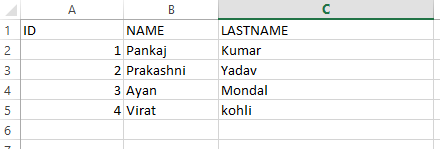Related Articles

# Reading and Writing data to excel file using Apache POI

• Last Updated : 17 Sep, 2017

Earlier in this post, we introduced Apache POI- a Java API useful for interacting with Microsoft office documents.
Now we’ll see how can we read and write to an excel file using the API.

Writing an excel file

Writing a file using POI is very simple and involve following steps:

1. Create a workbook
2. Create a sheet in workbook
3. Create a row in sheet
5. Repeat step 3 and 4 to write more data
 `// import statements``public` `class` `POIforgfgWrite {``public` `static` `void` `main(String[] args)``    ``{``        ``// Blank workbook``        ``XSSFWorkbook workbook = ``new` `XSSFWorkbook();`` ` `        ``// Create a blank sheet``        ``XSSFSheet sheet = workbook.createSheet(``"student Details"``);`` ` `        ``// This data needs to be written (Object[])``        ``Map data = ``new` `TreeMap();``        ``data.put(``"1"``, ``new` `Object[]{ ``"ID"``, ``"NAME"``, ``"LASTNAME"` `});``        ``data.put(``"2"``, ``new` `Object[]{ ``1``, ``"Pankaj"``, ``"Kumar"` `});``        ``data.put(``"3"``, ``new` `Object[]{ ``2``, ``"Prakashni"``, ``"Yadav"` `});``        ``data.put(``"4"``, ``new` `Object[]{ ``3``, ``"Ayan"``, ``"Mondal"` `});``        ``data.put(``"5"``, ``new` `Object[]{ ``4``, ``"Virat"``, ``"kohli"` `});`` ` `        ``// Iterate over data and write to sheet``        ``Set keyset = data.keySet();``        ``int` `rownum = ``0``;``        ``for` `(String key : keyset) {``            ``// this creates a new row in the sheet``            ``Row row = sheet.createRow(rownum++);``            ``Object[] objArr = data.get(key);``            ``int` `cellnum = ``0``;``            ``for` `(Object obj : objArr) {``                ``// this line creates a cell in the next column of that row``                ``Cell cell = row.createCell(cellnum++);``                ``if` `(obj ``instanceof` `String)``                    ``cell.setCellValue((String)obj);``                ``else` `if` `(obj ``instanceof` `Integer)``                    ``cell.setCellValue((Integer)obj);``            ``}``        ``}``        ``try` `{``            ``// this Writes the workbook gfgcontribute``            ``FileOutputStream out = ``new` `FileOutputStream(``new` `File(``"gfgcontribute.xlsx"``));``            ``workbook.write(out);``            ``out.close();``            ``System.out.println(``"gfgcontribute.xlsx written successfully on disk."``);``        ``}``        ``catch` `(Exception e) {``            ``e.printStackTrace();``        ``}``    ``}``}`

Reading an excel file is also very simple if we divide this in steps.

1. Create workbook instance from excel sheet
2. Get to the desired sheet
3. Increment row number
4. iterate over all cells in a row
5. repeat step 3 and 4 until all data is read
 `// import statements``public` `class` `POIforgfgRead {``public` `static` `void` `main(String[] args)``    ``{``        ``try` `{``            ``FileInputStream file = ``new` `FileInputStream(``new` `File(``"gfgcontribute.xlsx"``));`` ` `            ``// Create Workbook instance holding reference to .xlsx file``            ``XSSFWorkbook workbook = ``new` `XSSFWorkbook(file);`` ` `            ``// Get first/desired sheet from the workbook``            ``XSSFSheet sheet = workbook.getSheetAt(``0``);`` ` `            ``// Iterate through each rows one by one``            ``Iterator rowIterator = sheet.iterator();``            ``while` `(rowIterator.hasNext()) {``                ``Row row = rowIterator.next();``                ``// For each row, iterate through all the columns``                ``Iterator cellIterator = row.cellIterator();`` ` `                ``while` `(cellIterator.hasNext()) {``                    ``Cell cell = cellIterator.next();``                    ``// Check the cell type and format accordingly``                    ``switch` `(cell.getCellType()) {``                    ``case` `Cell.CELL_TYPE_NUMERIC:``                        ``System.out.print(cell.getNumericCellValue() + ``"t"``);``                        ``break``;``                    ``case` `Cell.CELL_TYPE_STRING:``                        ``System.out.print(cell.getStringCellValue() + ``"t"``);``                        ``break``;``                    ``}``                ``}``                ``System.out.println(``""``);``            ``}``            ``file.close();``        ``}``        ``catch` `(Exception e) {``            ``e.printStackTrace();``        ``}``    ``}``}`

Output:Sometimes you need to read a file at different location: Here’s how you can do it:

 `private` `static` `final` `String FILE_NAME``    ``= ``"C:\\Users\\pankaj\\Desktop\\projectOutput\\mobilitymodel.xlsx"``;``public` `static` `void` `write() ``throws` `IOException, InvalidFormatException``{``    ``InputStream inp = ``new` `FileInputStream(FILE_NAME);``    ``Workbook wb = WorkbookFactory.create(inp);``    ``Sheet sheet = wb.getSheetAt(``0``);``    ``........``}`

You can append to the existing file using following code:

 `private` `static` `final` `String FILE_NAME``    ``= ``"C:\\Users\\pankaj\\Desktop\\projectOutput\\blo.xlsx"``;``public` `static` `void` `write() ``throws` `IOException, InvalidFormatException``{``    ``InputStream inp = ``new` `FileInputStream(FILE_NAME);``    ``Workbook wb = WorkbookFactory.create(inp);``    ``Sheet sheet = wb.getSheetAt(``0``);``    ``int` `num = sheet.getLastRowNum();``    ``Row row = sheet.createRow(++num);``    ``row.createCell(``0``).setCellValue(``"xyz"``);``    ``.....``        ``..``        ``// Now this Write the output to a file``        ``FileOutputStream fileOut = ``new` `FileOutputStream(FILE_NAME);``    ``wb.write(fileOut);``    ``fileOut.close();``}`

This article is contributed by Pankaj Kumar. If you like GeeksforGeeks and would like to contribute, you can also write an article using contribute.geeksforgeeks.org or mail your article to contribute@geeksforgeeks.org. See your article appearing on the GeeksforGeeks main page and help other Geeks.